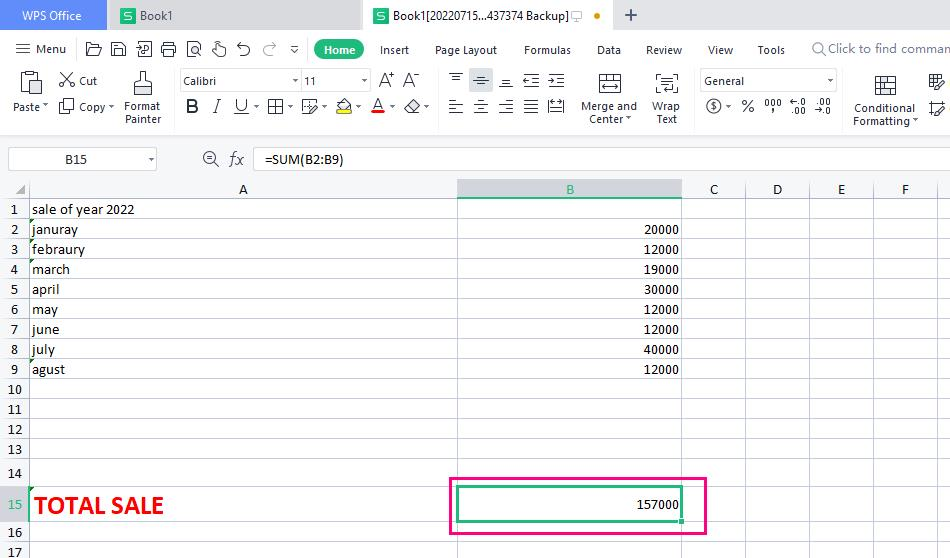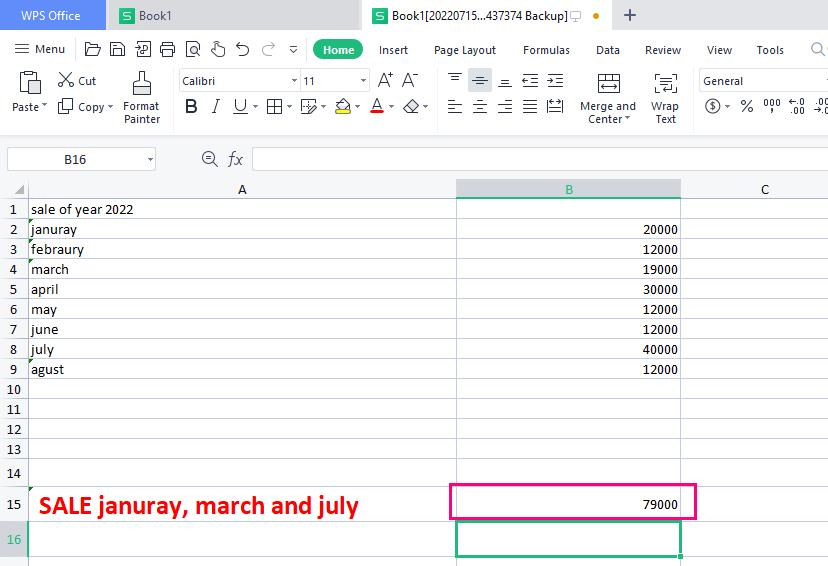# How to use sum function in excel

July 26, 2022
4.9K Views
0

A free Office suite fully compatible with Microsoft OfficeSUM Function in Excel is a part of math function. It can be used as a worksheet function in Excel and this function is used to count the number of cells that contain numbers. If a cell is empty or not numeric, it will be ignored. This article will explain how to use sum function in excel.

If you know how to use sum function in excel, the excel SUM function can be used to quickly add up the values contained in a range of cells. It falls within the Math and Trigonometry category of functions, and its input consists of typing =SUM followed by the values that are to be added up at the end. The function can take in values in the form of numbers, cell references, or range references as its parameters.

# How to use sum function in excel to sum range and sum entire column and row?

Sum Range:

1. After opening WPS spreadsheet select the cell where you want to sum the range.

2. Enter the formula in selected cell =SUM(B2:B9) in other way =sum(b2,b3,b4,b5,b6,b7,b8,b9) and press enter.

3. Once you press enter you will get the desired result means sum of chosen range in selected cell.Sum entire Column or Row:

To sum a whole column, you can alternatively use Excel's SUM function.

1. After opening WPS spreadsheet select the cell where you want to sum the Column or Row.

2. Enter the formula in selected cell =SUM(B:B) in order to sum the entire Row =sum(5:5) and press enter.

3. Once you press enter you will get the desired result means sum of whole column or row in selected cell.

# How to use sum function in excel to Sum Non-contiguous Cells?

To sum non-contiguous cells, you can use utilize Excel's SUM function. Non-contiguous refers to not being next to each other.

1. After opening WPS spreadsheet select the cell where you want to sum the contiguous cells.

2. Enter the formula in selected cell =SUM(b2,b4,b8).

3. Once you press enter you will get the desired result means sum of cell you have enter in formula..

# How to use sum function in excel without using sum word in formula?

Sum cell without using sum word:

1. After opening WPS spreadsheet select the cell where you want to sum the numbers.

2. Enter the formula in selected cell =b2+b3+b4+b5.

3. Once you press enter you will get the desired result means sum of mentioned cells.

This article provides an explanation of the features that are associated with how to use Sum Function in Excel to get the correct and quick result. If, on the other hand, you are interested in learning more about the WPS office, I would like to direct your attention to the official website of the WPS Academy. The free version of WPS Office, which can be downloaded from this website, enables users to make changes to a variety of file types, including PDFs, Word spreadsheets, and PowerPoint presentations.